# 作业要求一 （20分）

## (1）C高级第三次PTA作业（1）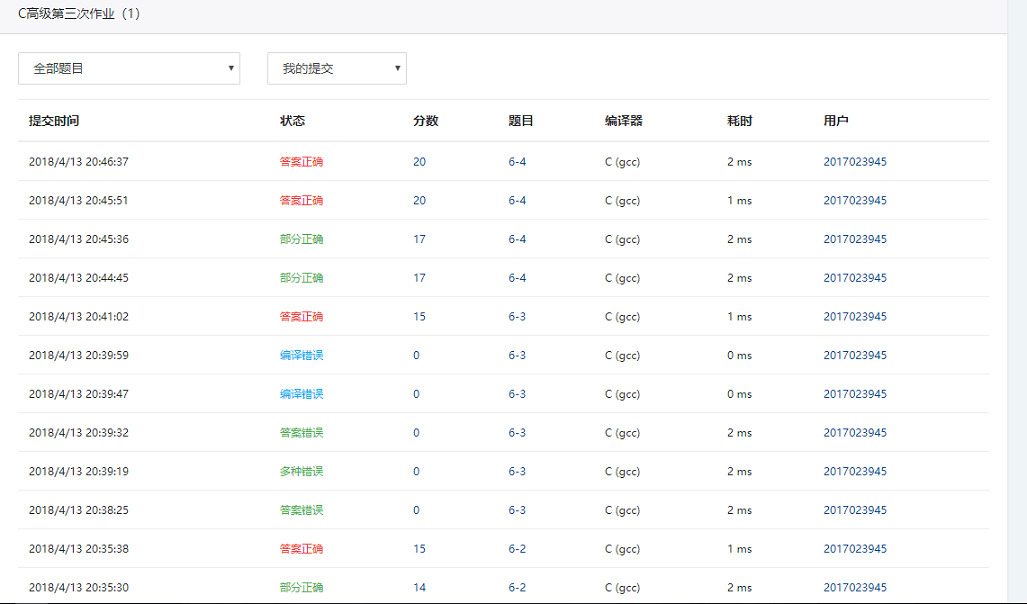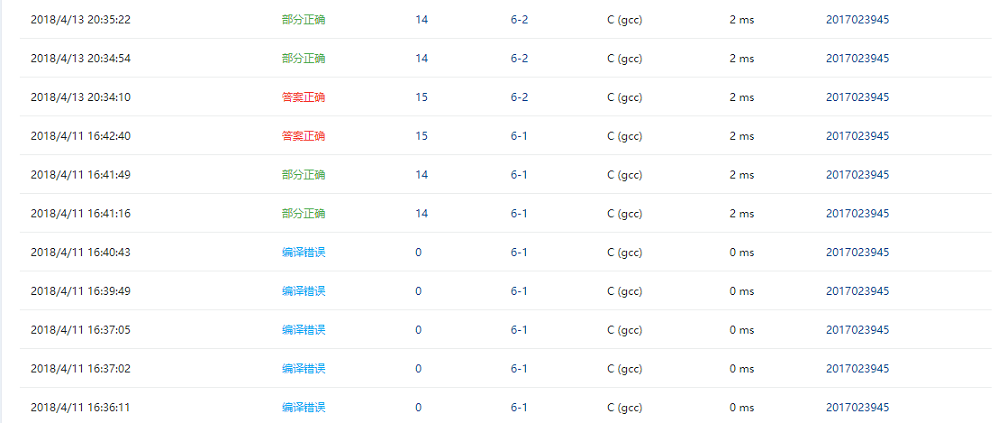## (2）一道编程题：

### 2.实验代码（2分）

#include <stdio.h>
int main ()
{
int a=201,b=3945;
int *p = (int *)malloc((a*b) *sizeof(int));
int *q = (int *)malloc((a*b) *sizeof(int));
int i=0,j;
for(i=0;i<(a*b);i++)
{
p[i] = i+1;
}
for(i=0;i<(a*b);i++)
{
for(j = i+1;j<=(a*b);j++)
{
if((p[i] !=1) && ( p[j] != 1) ){
if(p[j]%p[i] ==0)
{
p[j] = 1;
}
}
}
}
j=0;
for(i=0;i<(a*b);i++)
{
if(p[i] != 1)
{
printf(" %d",p[i]);
j++;
}
if(j == 5)
{
printf("\n");
j=0;
}
}
}


# 作业要求二（65分）

## 6-1 输出月份英文名

### 2.实验代码（2分）

#### 部分代码：

 char *getmonth( int n )
{
switch(n)
{
case 1:return "January";
case 2:return "February";
case 3:return "March";
case 4:return "April";
case 5:return "May";
case 6:return "June";
case 7:return "July";
case 8:return "August";
case 9:return "September";
case 10:return "October";
case 11:return "November";
case 12:return "December";
default:return NULL;
}
}


## 6-2 查找星期（15 分）

### 1.设计思路（6分）

(2)流程图（4分）### 2.实验代码（2分）

#### 部分代码

int getindex( char *s )
{
int c=-1,a;
char *week={"Sunday","Monday","Tuesday","Wednesday","Thursday","Friday","Saturday"};
for(a=0;a<7;a++)
{
if(strcmp(s,week[a])==0)
{
c=a;
break;
}
}
return c;
}


## 6-3 计算最长的字符串长度（15 分）

(1)主要描述题目算法（2分）

max。

(2)流程图（4分）

### 2.实验代码（2分）

#### 部分代码

int max_len( char *s[], int n )
{
int a,c=0,b,q={0};
for(a=0;a<n;a++)
{
for(b=0;*(*(s+a)+b)!='\0';b++)
{}
q[a]=b;
}
c=q;
for(a=0;a<n;a++)
{
if(q[a]>c)
{
c=q[a];
}
}
return c;
}


## 6-4 指定位置输出字符串（20 分）

(1)主要描述题目算法（2分）

### 2.实验代码（2分）

#### 部分代码

char *match( char *s, char ch1, char ch2 )
{
int a,b;
for(a=0;*(s+a)!='\0';a++)
{
if(*(s+a)==ch1)
{
char *c=  &s[a];
for(b=a;(*(s+b)!=ch2)&(*(s+b)!='\0');b++)
{
printf("%c",*(s+b));
}
if(*(s+b)!='\0')
printf("%c",*(s+b));
printf("\n");
return c;
}
}
printf("\n");
return s+a;
}


(s+i),程序运行成功。

## 6-1 奇数值结点链表（20 分）

(1)主要描述题目算法（2分）

(2)流程图（4分）

### 2.实验代码（2分）

#### 部分代码

    struct ListNode *readlist()
{
int number;
struct ListNode *p = NULL,*head = NULL,*tail = NULL;
scanf("%d",&number);
while(number!=-1 && number>0 )
{
p = (struct ListNode*)malloc(sizeof(struct ListNode));
p->data = number;
{
} else
{
tail->next = p;
}
tail = p;
scanf("%d",&number);
}
{
return NULL;
}
tail->next = NULL;
}
struct ListNode *getodd( struct ListNode **L )
{
struct ListNode *p = *L,*head1 = NULL,*r = NULL,*r1 = NULL,*L1 = NULL;
while(p!=NULL&&p->data>0)
{
if(p->data%2!=0)
{
{
} else
{
r->next = p;
}
r = p;
} else
{
if(L1 ==NULL)
{
L1 = p;
} else
{
r1->next = p;
}
r1 = p;
}
p = p->next;
}
{
return NULL;
} else
{
r->next = NULL;
}
if(L1==NULL)
{
*L = NULL;
} else
{
r1->next = NULL;
*L = L1;
}
}


### 3.本题调试过程碰到问题及解决办法（4分）

     课程学习过程中，对链表的结构，表示很不清晰。
改正方法：询问同学后才明白。


## (1）C高级第三次PTA作业（2）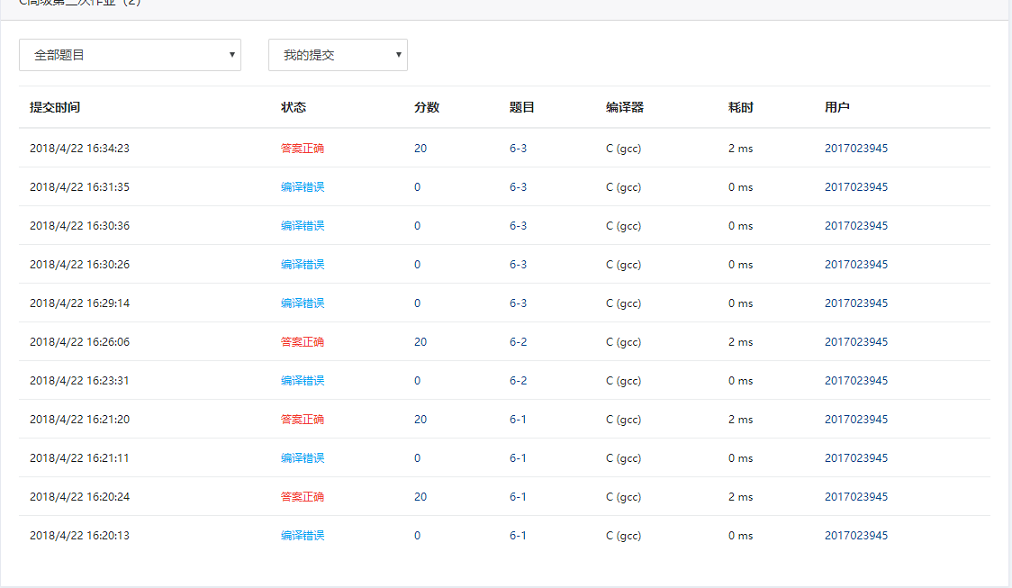## 6-1 奇数值结点链表

### 1.设计思路（6分）

(1)主要描述题目算法（2分）

(2)流程图（4分）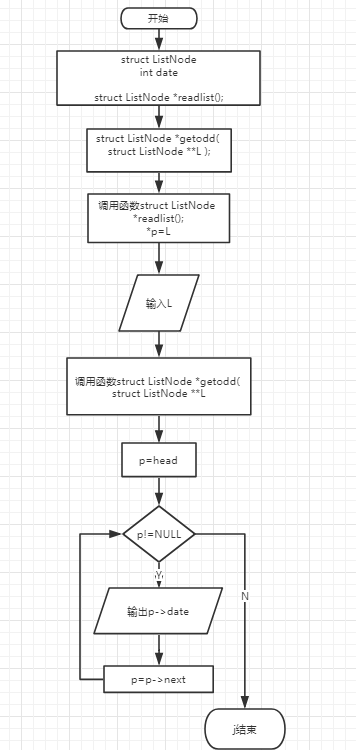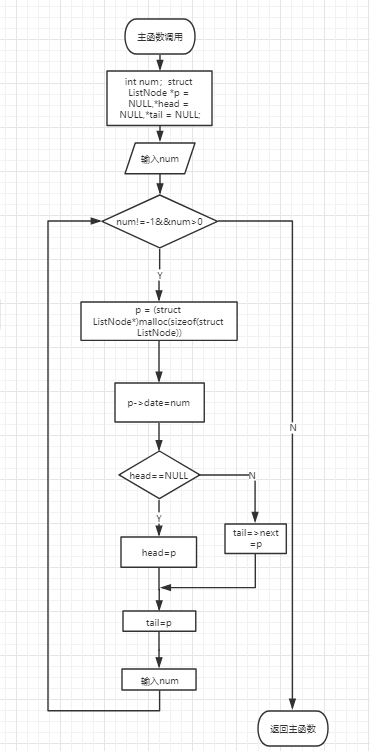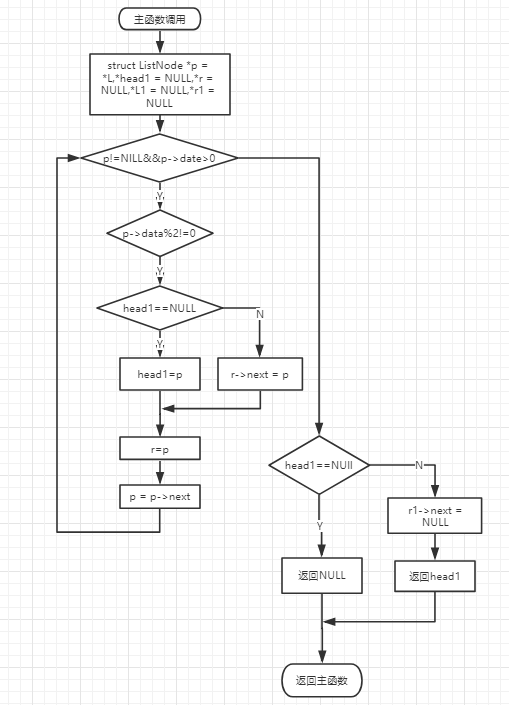### 2.实验代码（2分）

struct stud_node *createlist()
{
int num,score;
char name;
p = (struct stud_node*)malloc(sizeof(struct stud_node));
scanf("%d",&num);

while (num != 0)
{
p = (struct stud_node *)malloc (sizeof (struct stud_node));
scanf ("%s %d", p->name, &p->score);
p->num = num;

else
tail->next = p;
tail = p;
scanf ("%d", &num);
}
}
struct stud_node *deletelist( struct stud_node *head, int min_score )
{
while(L!=NULL)
{
if(L->score>=min_score)
{
{
} else
{
tail1->next = L;
}
tail1 = L;
} else
{

}
L=L->next;
}
return NULL;
} else
{
tail1->next =NULL;
}
}


## 6-2 学生成绩链表处理（20 分）

(1)主要描述题目算法（2分）

(2)流程图（4分）

### 2.实验代码（2分）

struct ListNode *mergelists(struct ListNode *list1, struct ListNode *list2)
{
struct ListNode *p1=list1;
int length=0;
int array;
for(p1=list1;p1!=NULL;p1=p1->next)
{
array[length] = p1->data;
length++;
}
p1=list2;
for(;p1!=NULL;p1=p1->next)
{
array[length] = p1->data;
length++;
}
int i,j,t;
for(i=0;i<length-1;i++)
{
for(j=i+1;j<length;j++)
{
if(array[j]<array[i])
{
t =array[j];
array[j]=array[i];
array[i] = t;
}
}
}
i=0;
while(i<length)
{
q = (struct ListNode *)malloc(sizeof(struct ListNode));
q->data = array[i];
{
} else
{
tail1->next = q;
}
tail1 = q;
tail1->next = NULL;
i++;
}
}


## 6-3 链表拼接（20 分）

(1)主要描述题目算法（2分）

### 2.实验代码（2分）

  struct ListNode *mergelists(struct ListNode *list1, struct ListNode *list2)
{
struct ListNode *p1=list1;
int length=0;
int array;
for(p1=list1;p1!=NULL;p1=p1->next)
{
array[length] = p1->data;
length++;
}
p1=list2;
for(;p1!=NULL;p1=p1->next)
{
array[length] = p1->data;
length++;
}
int i,j,t;
for(i=0;i<length-1;i++)
{
for(j=i+1;j<length;j++)
{
if(array[j]<array[i])
{
t =array[j];
array[j]=array[i];
array[i] = t;
}
}
}
i=0;
while(i<length)
{
q = (struct ListNode *)malloc(sizeof(struct ListNode));
q->data = array[i];
{
} else
{
tail1->next = q;
}
tail1 = q;
tail1->next = NULL;
i++;
}
}


# 要求三、学习总结和进度（15分）

## 1、总结两周里所学的知识点，回答下列问题？（用自己的话表达出你的理解，网上复制粘贴没有分数）（5分)

### （2）将C高级第三次PTA作业（1）任何一个题目改为使用二级指针对指针数组进行操作。

 char *getmonth( int n ) {
char *month = {"January","February","March","April","May","June","July","August","September","October","November","December"};
char **p = &month;
char *pc = '\0';
if(n>0 && n<=12)
{
pc = *(p+n-1);
}
return pc;
}


## 2、将PTA作业的源代码使用git提交到托管平台上，要求给出上传成功截图和你的git地址。（3分）git地址:(https://git.coding.net/smj031915/dexqdcczy.git)

## 4、请用表格和折线图呈现你本周（4/9 8:00~4/23 8:00）的代码行数和所用时间、博客字数和所用时间（3分）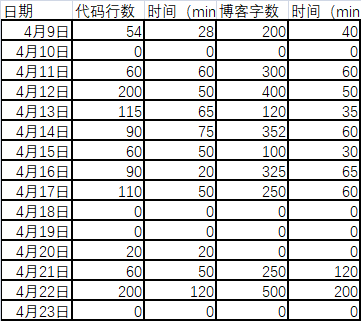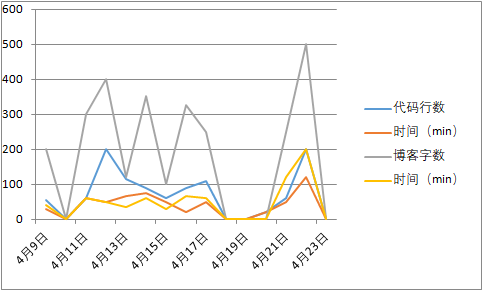posted @ 2018-04-14 22:13  莯菸  阅读(252)  评论(12编辑  收藏  举报